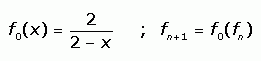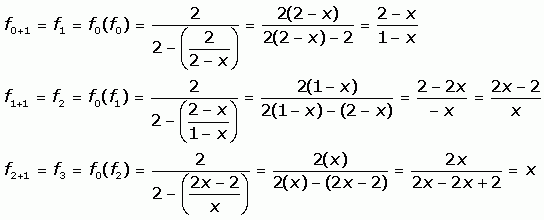Question from RJ, a high school teacher: My son asked me this question and I am not sure about it so if you could help me solve it I would greatly appreciate it. The question is : Let f0(x) = 2/2-x and fn+1 = f0 o fn for n greater than or equal to 0. Find a formula for fn and prove it by mathematical induction. Recall that o represents function composition. i.e., (f o g)(x) = f(g(x)). Hi RJ. Whenever you are trying to come up with a formula or whenever starting out a mathematical induction proof, it is worthwhile to try the first few values of n to see what is happening. So starting with the function definition:Let's look at the first few values of fn (x):So this function cycles. The proof is almost done already, so I'll leave the rest to you. Stephen La Rocque.> RJ wrote back Hi, I understand everything you showed me in the answer, but how does that show me the proof by mathematical induction. I don’t understand, all it seems like is that you are plugging in one equation for the other. I'm still a little confused. So if you could give me a little more insight I would appreciate it. Thanks What Sue did was to show that fo(x) = 2/(2-x) f1(x) = (2-x)/(1-x) f2(x) = (2x - 2)/x and f3(x) = x From here it follows that f4 (x) = fo o f3 (x) = fo(x) = 2/(2-x) and it follows that f5(x) = f1(x) f6(x) = f2(x) f7(x) = f3(x) and the pattern repeats. The essence of the induction argument is to be more formal with the clause the pattern repeats. I am going to change the indexing so that it starts at 1 rather than 0. Let gn = fn-1for n = 1, 2, ... For each n there are integers k and i with i = 1, 2, 3 or 4 so that n = 4k + i. The pattern I see and want to prove is that for each k g4k+1(x) = 2/(2-x) g4k+2(x) = (2-x)/(1-x) g4k+3(x) = (2x - 2)/x and g4k+4(x) = x I am going to prove this using induction on k. Sue proved this for k = 0. This is the first step in the induction argument. I want to show that the pattern repeats. To do this I assume that I have proved this for some particular value of k and I want to verify it is true for the next value of k. So I am assuming the 4 equations above are true for some particular value of k but not necessarily for any larger k. Using the definition in the problem g4(k+1)+1(x) = g(4k+4)+1 (x) = f0(g4k+4(x)) = f0(x) = 2/(2-x) In a similar fashion you can show that g4(k+1)+2(x) = (2-x)/(1-x) g4(k+1)+3(x) = (2x - 2)/x and g4(k+1)+4(x) = x Hence the pattern does hold for k+1. Thus, by induction the formula I found for fn is valid for all n = 0, 1, 2, ... Penny